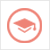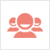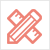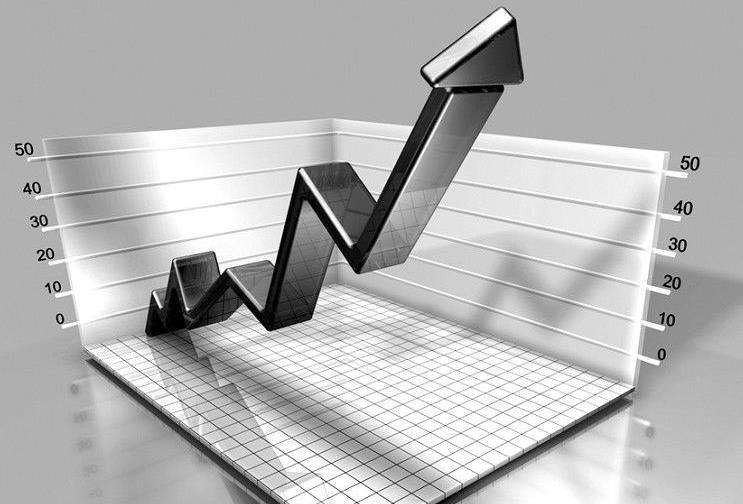﻿ Essay范文-The application of mathematical theory in modern economy-51Due留学教育

#### 服务承诺资金托管原创保证实力保障24小时客服使命必达

#### 关于我们

51Due提供Essay，Paper，Report，Assignment等学科作业的代写与辅导，同时涵盖Personal Statement，转学申请等留学文书代写。51Due将让你达成学业目标51Due将让你达成学业目标51Due将让你达成学业目标51Due将让你达成学业目标

#### 名企实习积累工作经验多元化文化交流专业实操技能建立人际资源圈

# The application of mathematical theory in modern economy

2019-12-17 来源: 51Due教员组 类别: Essay范文Mathematics is the basis of science and technology and has certain intellectual value and application value. In the era of knowledge economy, the fields of technology and economic life are widely permeated with mathematical theory.

The goal of economic development is to reduce the production cost, improve the production efficiency and increase the production income, so in the process of economic management, we need to pay attention to the cost, income and profit. Coordinate production and sales to maximize returns with minimal investment. Using mathematical theory in modern economy can support the development of economic management.

Mathematics is a basic discipline, which is relatively rigorous. It has begun to widely use mathematical theories in economic activities, which can reduce the negative impact of uncertainty on the economy. Through the establishment of mathematical models to analyze various economic factors, and then determine the optimal conditions through calculation, to provide support for enterprise decision-making. Mathematical theory can express the relationship between cash flow and performance indicators of enterprises and predict the risks that enterprises may face in the actual operation process. In the primary stage of development, mathematical theory is only widely used in political economy, among which elementary mathematical knowledge and quantitative analysis are commonly used. Later, mathematical theory and economic theory were constantly integrated. In the economic research process began to make more use of mathematical theory, for example in the economy began to make more and more use of calculus and probability and statistics and other advanced mathematical theory. The current mathematical theory and modern economic theory have been closely combined, learning mathematical theory is conducive to the study of economic knowledge, the use of mathematical tools can clearly reflect the problems in economic development, the new mathematical theory is conducive to the study of cost calculation and other aspects. Using mathematical theory modeling, enterprise managers can strictly control the cost of each operation link to ensure the maximization of profits. Mathematical theory has diversified characteristics and can guide the development of economy. As a tool, mathematics can solve practical problems. Enterprises should strengthen economic research and determine variable relationships from the perspective of data when dealing with practical problems, so as to improve the correctness of economic decisions.

Modern economics to optimize the use of social resources, and giving people the productive relations to integration, using various means to deal with people and the value of the relationship between material, reasonable application of mathematical theory, can be analyzed and summarized the economic behavior of involved data, to ensure implementation plan optimization, make the resource utilization efficiency is improved.

When constructing mathematical models, we should closely combine economic management data with mathematical modeling, combine the actual situation with theoretical knowledge, and analyze the actual situation according to the characteristics of modern economics.

When analyzing the properties of data variables in modern economic management, it is necessary to construct the conceptual model in mathematical theory, which is beneficial to derive data results, otherwise, the economic connotation of mathematics cannot be analyzed out. When dealing with the problem of economic management, we should combine the data and concepts of the mathematical model to grasp the Angle of economic quantity and not be divorced from the economic nature of the mathematical model. To study the statistical content in modern economic management, it is necessary to extract the data from the mathematical model, and the selected data play an important role, so that the model needs to choose the data and make the data accuracy continuously improve. When studying the generation state of modern economic management data, the spatio-temporal limit of data should be considered in the process of data selection, so that a suitable mathematical model can be selected. Specific values can be obtained through mathematical model calculation, and relevant economic concepts should be combined in the analysis of values, so as to formulate economic plans based on these results, which can guide specific economic behaviors.

Establishing a scientific and reasonable relationship between production and sales is conducive to the stable development of the enterprise. Using mathematical theory can guide the output of enterprises scientifically and ensure the scientificity and accuracy of enterprise decision-making. For example, if the monthly sales volume of an enterprise is 1 million and the gross profit rate is 8%, calculate the gross profit obtained every month. When solving the problem, we can use the mathematical theory knowledge, use the mathematical formula to transform the problem, determine the proportional relationship between gross profit and sales, sales × gross profit = gross profit, 100×8%= 80,000 yuan.

Business costs include fixed and variable costs, which are depreciation, executive salaries, and promotional expenses. Variable costs are uncertain and need to be changed in combination with reality. Linear algebra knowledge of mathematical theory can be used to show the relationship between production and cost. For example, fixed cost + variable cost = total cost, and single cost × volume + fixed cost = total cost. Using the mathematical theory of the function can be expressed explicitly. For example, the total cost is y, the total production is x, the variable cost per unit product is a, and the specified cost is c, which can be expressed as y=ax+c. This shows that mathematical knowledge can be used to calculate the cost of enterprises and promote the stable development of enterprises.

To sum up, the application of mathematical theory in modern economy has great advantages. Mathematical modeling and mathematical theory can transform complex economic knowledge and more easily calculate the impact of variables on costs and benefits, so as to guide economic decision-making of enterprises and promote sustainable development of enterprises.

51due留学教育原创版权郑重声明：原创essay代写范文源自编辑创作，未经官方许可，网站谢绝转载。对于侵权行为，未经同意的情况下，51Due有权追究法律责任。主要业务有essay代写、assignment代写、paper代写、作业代写服务。

51due为留学生提供最好的作业代写服务，亲们可以进入主页了解和获取更多代写范文 提供作业代写服务，详情可以咨询我们的客服QQ：800020041。